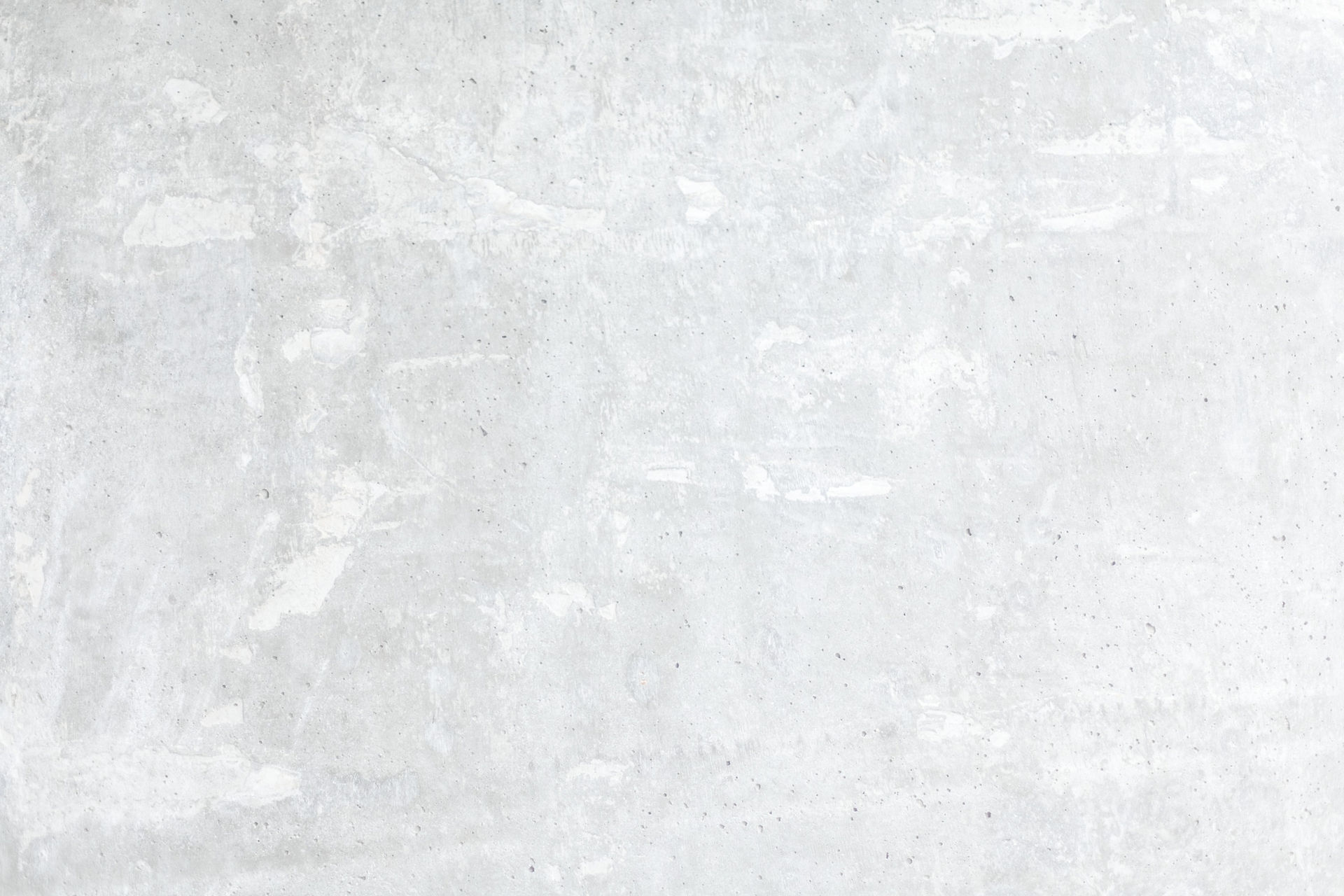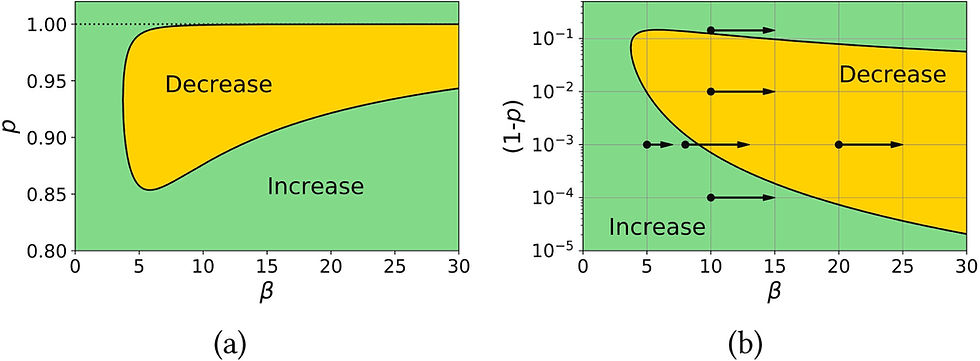top of pageSearch
• Bill Jin

# How do specific surface area and particle size distribution change when granular media dissolve?Fig. 1. Incremental change in specific surface during dissolution for a granular medium with two particle sizes having a size ratio of β, given that the number fraction of smaller particles is p: (a) shows the curve that distinguishes the regions where the change is positive or negative, with a dotted line at p=1 for comparison; (b) shows the same curve, with the quantity (1−p) on the y-axis. The filled circles in (b) indicate the starting points of the systems considered in Fig. 2, and the arrows indicate the direction of the path taken.Fig. 2. Predictions of the evolution in scaled specific surface area (S=s(ϵ)∕s(0)) during uniform dissolution of a bimodal particle size distribution. The left plot shows the influence of the number fraction of the smaller particles for a fixed initial size ratio of β=10. The right plot shows the influence of that initial size ratio for a fixed number fraction p=0.999 of the smaller particles. The initial behavior, whether increasing, decreasing, or remaining relatively constant, is indicated by the diagram in Fig. 1.

2021-02_SSA and PSD when particle dissov
.